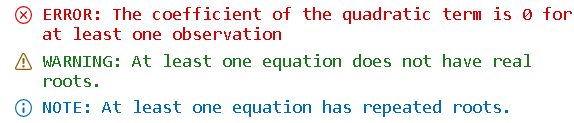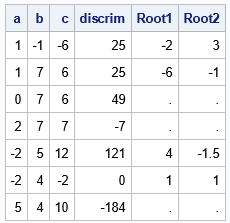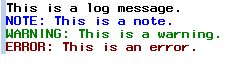I previously discussed how to use the PUTLOG statement to write a message from the DATA step to the log in SAS. The PUTLOG statement is commonly used to write notes, warnings, and errors to the log. This article shows how to use the PRINTTOLOG subroutine in SAS IML software to write messages to the log. The PRINTTOLOG subroutine was released in 2020 in SAS IML on SAS Viya. It runs in PROC IML and in the iml action on Viya. The PRINTTOLOG subroutine is not part of SAS 9.4, but I show how to construct a SAS/IML module for SAS 9.4 that has the same syntax and functionality.

### The PrintToLog subroutine in SAS IML on Viya

The syntax of the subroutine is
call PrintToLog(msg, flag);
The flag parameter is optional. If you omit that argument, the message is displayed in the log. If you use the flag parameter, the valid values are 0, 1, and 2:

• If flag is 0, the string "NOTE: " is prepended to the message. For example, call PrintToLog("This is a note.", 0);.
• If flag is 1, the string "WARNING: " is prepended to the message. For example, call PrintToLog("This is a warning.", 1);.
• If flag is 2, the string "ERROR: " is prepended to the message. For example, call PrintToLog("This is an error.", 2);.

I will illustrate the routine by using an IML version of the program that I used in my previous post about the PUTLOG statement. The input to the program is a three-column matrix. Each row of the matrix contains the coefficients (a, b, c) for the quadratic equation a x2 + b x + 3 = 0. The program is vectorized. It uses the discriminant of the quadratic equation to determine whether the quadratic equation has any real roots. If so, the program uses the quadratic formula to find the roots. To demonstrate the PrintToLog function, the program does the following:

• Display an ERROR if any coefficient of the quadratic term is 0.
• Display a WARNING if any quadratic equation does not have any real roots.
• Display a NOTE if any quadratic equation has a repeated root.
```proc iml; /* NOTE: The PrintToLog subroutine is available in SAS IML in Viya. It is not available as a built-in subroutine for SAS/IML in SAS 9.4. */ Q = { 1 -1 -6, /* each row is (a,b,c) */ 1 7 6, 0 7 6, 2 7 7, -2 5 12, -2 4 -2, 5 4 10}; a = Q[,1]; b = Q[,2]; c = Q[,3]; Root1 = j(nrow(a), 1, .); /* initialize roots to missing */ Root2 = j(nrow(a), 1, .); /* initialize roots to missing */   if any(a=0) then call PrintToLog("The coefficient of the quadratic term is 0 for at least one observation", 2); /* display ERROR */   discrim = b##2 - 4*a#c; if any(discrim < 0) then call PrintToLog("At least one equation does not have real roots.", 1); /* display WARNING */ if any(discrim=0) then call PrintToLog("At least one equation has repeated roots.", 0); /* display NOTE */   idx = loc(discrim >= 0 && a^=0); if ncol(idx)>0 then do; Root1[idx] = (-b[idx] - sqrt(discrim[idx])) / (2*a[idx]); Root2[idx] = (-b[idx] + sqrt(discrim[idx])) / (2*a[idx]); end; print a b c discrim Root1 Root2;```

The SAS log contains the following message, which is color-coded according to whether the message is an error (red color), a warning (green color), or a note (blue color).For completeness, the output of the program is shown below.### A PrintToLog module for SAS 9.4

Although the PrintToLog subroutine is not a built-in subroutine in SAS 9.4, you can define a module that has the same functionality. The definition uses two SAS/IML techniques and one macro trick:

The following SAS macro defines a SAS/IML module in SAS 9.4, but not in SAS Viya. Thus, you can run this macro in any PROC IML program. If the program runs in Viya (where the PrintToLog subroutine is already defined), the macro does nothing. If the program runs in SAS 9.4, the macro defines a PrintToLog module that you can use to write messages to the log.

```/* Check the SYSVER macro to see if SAS 9.4 is running. In SAS Viya, the macro is empty and does nothing. In SAS 9.4, the macro defines a function that emulates the PrintToLog call. The syntax is as follows: call PrintToLog("This is a log message."); call PrintToLog("This is a note.", 0); call PrintToLog("This is a warning.", 1); call PrintToLog("This is an error.", 2); */ %macro DefinePrintToLog; %if %sysevalf(&sysver = 9.4) %then %do; start PrintToLog(msg,errCode=-1); if errCode=0 then prefix = "NOTE: "; else if errCode=1 then prefix = "WARNING: "; else if errCode=2 then prefix = "ERROR: "; else prefix = ""; stmt = '%put ' + prefix + msg + ';'; call execute(stmt); finish; %end; %mend;   /* this program runs in SAS 9.4 or in SAS Viya */ proc iml; %DefinePrintToLog; call PrintToLog("This is a log message."); call PrintToLog("This is a note.", 0); call PrintToLog("This is a warning.", 1); call PrintToLog("This is an error.", 2);```The output is shown for SAS 9.4, where the %DefinePrintToLog macro defines the SAS/IML module.

### Summary

The PRINTTOLOG statement is way for SAS IML programmers to write messages to the log. In SAS Viya, this subroutine is built-in and can be used from PROC IML or from the iml action. This article shows how to define a module for PROC IML in SAS 9.4 that has similar functionality.

Share